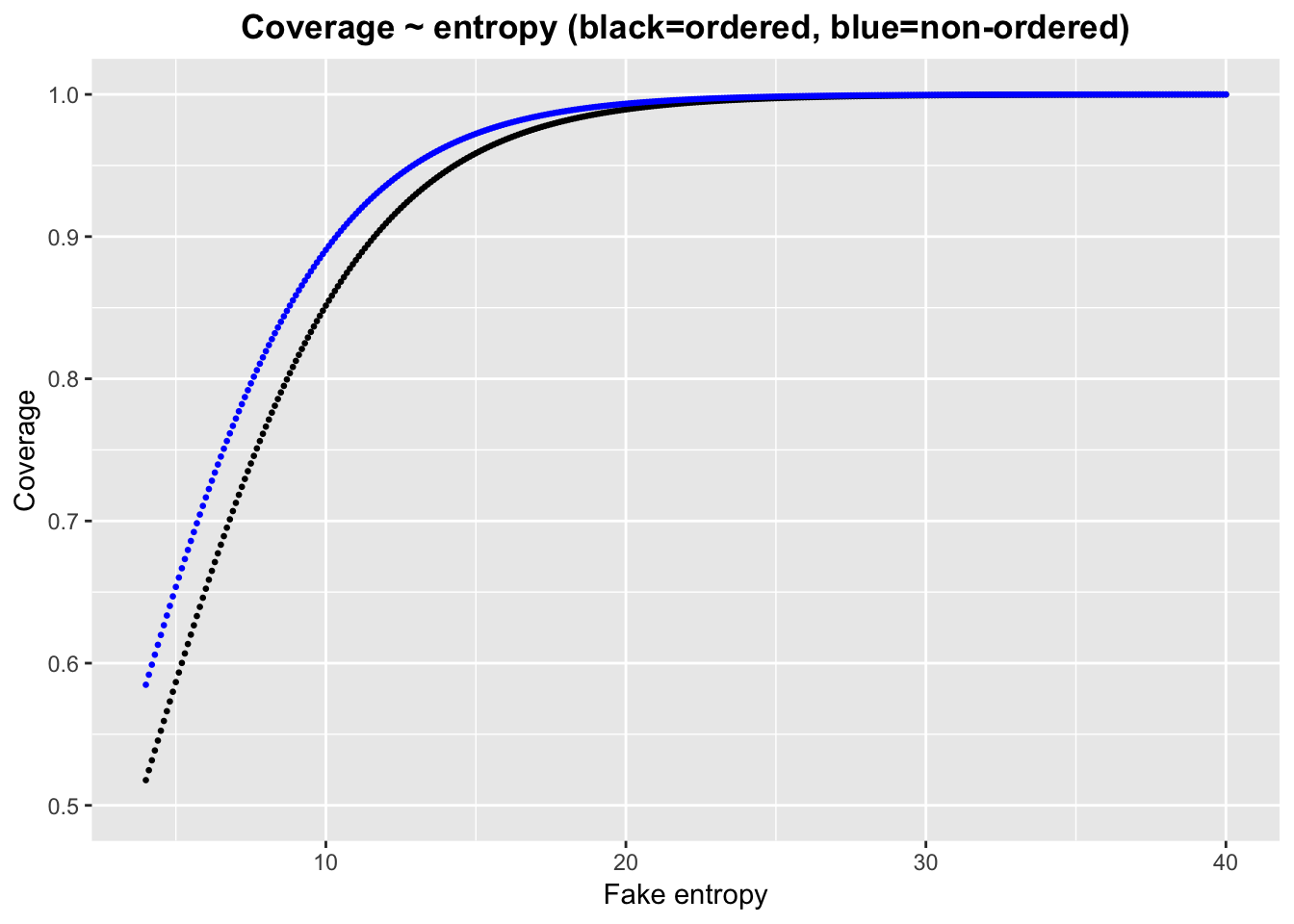#### Read in data/ data prep:

``````library(data.table)
setwd("/Users/anna/PhD")
cs <- read.table("cs for diff nsnps")
cs <- as.data.table(cs)
cs_ord <- cs[order=="TRUE"] # separate ordered and non-ordered simulations
cs_noord <- cs[order=="FALSE"]
cs_ord\$covered <- as.numeric(cs_ord\$covered) # has dim 200,000*9
cs_noord\$covered <- as.numeric(cs_noord\$covered) # has dim 200,000*9
cs_ord <- cs_ord[,-1] # remove order column
cs_noord <- cs_noord[,-1]
``````##    thr      size nvar covered  entropy    N  OR nsnps
## 1: 0.6 0.6094180   10       1 5.306601 5000 1.1   100
## 2: 0.6 0.6069801   32       0 4.859282 5000 1.1   100
## 3: 0.6 0.6598043    1       1 5.843130 5000 1.1   100
## 4: 0.6 0.7162386    1       1 6.000415 5000 1.1   100
## 5: 0.6 0.6133004    4       0 5.491237 5000 1.1   100
## 6: 0.6 0.6057816   10       1 5.310640 5000 1.1   100``````
``head(cs_noord)``
``````##    thr      size nvar covered  entropy    N  OR nsnps
## 1: 0.6 0.6231312   38       1 5.306601 5000 1.1   100
## 2: 0.6 0.6068766   54       0 4.859282 5000 1.1   100
## 3: 0.6 0.8186720   46       1 5.843130 5000 1.1   100
## 4: 0.6 0.7827822   14       1 6.000415 5000 1.1   100
## 5: 0.6 0.7888346   59       1 5.491237 5000 1.1   100
## 6: 0.6 0.6027625   49       0 5.310640 5000 1.1   100``````

The ‘cs’ dataset contains information for 400,000 credible set simulations with varying combinations of OR, threshold, N and nsnps values.

Sampled OR values: 1, 1.05, 1.1, 1.2, 1.3.

Sampled thr values:0.5,0.6,0.7,0.8,0.9.

Sampled N values: 1000, 2000, 3000, 4000, 5000.

Sampled nsnps values: 100, 300, 500, 1000.

#### Individual effect of each variable

Logistic models were fit with each variable as the sole predictor. Fake data was then created for each variable, and the corresponding logistic model used to predict coverage for the fake data.

``````xent <- glm(covered~entropy, data=cs_ord, family='binomial')
xthr <- glm(covered~thr, data=cs_ord, family='binomial')
xsize <- glm(covered~size, data=cs_ord, family='binomial')
xnsnps <- glm(covered~nsnps, data=cs_ord, family='binomial')
xN <- glm(covered~N, data=cs_ord, family='binomial')
xnvar <- glm(covered~nvar, data=cs_ord, family='binomial')
xOR <- glm(covered~OR, data=cs_ord, family='binomial')

fake_ent <- data.frame(entropy=seq(4,40,by=0.1))
fake_ent\$pred <- predict(xent,newdata=fake_ent,family=binomial,type="response")

fake_thr <- data.frame(thr=seq(0.5,1,by=0.01))
fake_thr\$pred <- predict(xthr,newdata=fake_thr,family=binomial,type="response")

fake_size <- data.frame(size=seq(0.5,1,by=0.01))
fake_size\$pred <- predict(xsize,newdata=fake_size,family=binomial,type="response")

fake_nsnps <- data.frame(nsnps=seq(100,1000,by=1))
fake_nsnps\$pred <- predict(xnsnps,newdata=fake_nsnps,family=binomial,type="response")

fake_N <- data.frame(N=seq(1000,5000,by=1))
fake_N\$pred <- predict(xN,newdata=fake_N,family=binomial,type="response")

fake_nvar <- data.frame(nvar=seq(1,843,by=1))
fake_nvar\$pred <- predict(xnvar,newdata=fake_nvar,family=binomial,type="response")

fake_OR <- data.frame(OR=seq(1,1.5,by=0.01))
fake_OR\$pred <- predict(xOR,newdata=fake_OR,family=binomial,type="response")``````

The same method was implemented for the non-ordered data for comparison

``````yent <- glm(covered~entropy, data=cs_noord, family='binomial')
ythr <- glm(covered~thr, data=cs_noord, family='binomial')
ysize <- glm(covered~size, data=cs_noord, family='binomial')
ynsnps <- glm(covered~nsnps, data=cs_noord, family='binomial')
yN <- glm(covered~N, data=cs_noord, family='binomial')
ynvar <- glm(covered~nvar, data=cs_noord, family='binomial')
yOR <- glm(covered~OR, data=cs_noord, family='binomial')

fake_ent\$pred1 <- predict(yent,newdata=fake_ent,family=binomial,type="response")
fake_thr\$pred1 <- predict(ythr,newdata=fake_thr,family=binomial,type="response")
fake_size\$pred1 <- predict(ysize,newdata=fake_size,family=binomial,type="response")
fake_nsnps\$pred1 <- predict(ynsnps,newdata=fake_nsnps,family=binomial,type="response")
fake_N\$pred1 <- predict(yN,newdata=fake_N,family=binomial,type="response")
fake_nvar\$pred1 <- predict(ynvar,newdata=fake_nvar,family=binomial,type="response")
fake_OR\$pred1 <- predict(yOR,newdata=fake_OR,family=binomial,type="response")``````

### Results

#### EntropyBoth curves plateau as the entropy value reaches approximately 20. This value represents a high disorder system, whereby one snp takes a huge proportion of the posterior probability. On analysis of the ordered data, there were many (~8%) cases where \(size=1\), \(nvar=1\) and \(covered=TRUE\), representing a system where one snp (the CV) has all the posterior probability. Conversely, in the non-ordered system, there were many instances of \(size=1\), but few with \(nvar=1\) (as this would only be the case when the first snp has all the posterior probability).

For any entropy value, the coverage for non-ordered sets is higher than that for ordered sets. This does not sound right as we know there are instances of over and undercoverage for different values of OR.

We know that entropy depends on OR, see below for seperate plots for each value of OR. Since they are all so different, seems silly to ‘squish’ them all into one plot. Idea: Could also include OR (and interaction term?) in the glm.

Ordered: